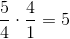## Example Questions

### Example Question #1 : How To Divide Decimals

What is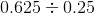?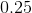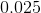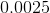Explanation:

0.625 is equal to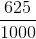, while .25 is equal to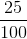. Dividing by fractions is the same as multiplying by the reciprocal of the divisor. Thus,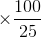which equals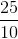, which simplifies to 2.5.

### Example Question #1 : How To Divide Decimals

Divide: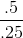Explanation:

Eliminate the decimal place on the denominator by moving the decimal place 2 spaces to the right.  Follow suit for the numerator.  The decimal place should also move the same number of units to the right.  Evaluate.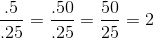### Example Question #1 : How To Divide Decimals

Divide: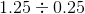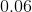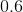Explanation:

Rewriteso that both are in fraction form.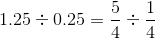Convert the division sign to multiplication, and take the reciprocal of the 2nd term.